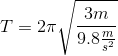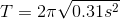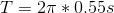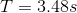# High School Physics : Using Pendulum Equations

## Example Questions

### Example Question #1 : Using Pendulum Equations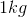mass on a pendulum is dropped from rest. It is observed that the period of the oscillation is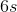. What is the length of the string?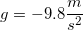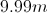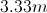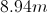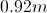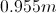Explanation:

The equation for the period of a pendulum is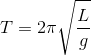.

The mass on the pendulum is irrelevant when calculating the relationship between period and string length, as the only variables in the equation are the length of the string and gravity.

Since we are given the period and the constant for gravity, we can solve for the string length.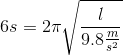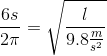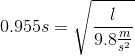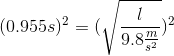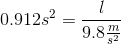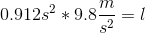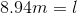### Example Question #2 : Using Pendulum Equations

A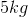mass on a pendulum is dropped from rest. If the length of the string is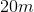, what is the period of oscillation?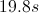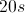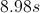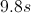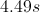Explanation:

The equation for the period of a pendulum is.

The mass on the pendulum is irrelevant when calculating the relationship between period and string length, as the only variables in the equation are the length of the string and gravity.

Since we are given the string length and the constant for gravity, we can solve for the period.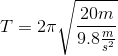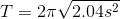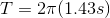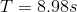### Example Question #3 : Using Pendulum Equations

A pendulum of length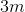is attached to a mass of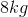. As it swings back and forth, what is the period?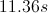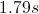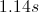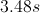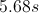Explanation:

The formula for period of a pendulum is: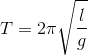We are given the length of the string and the acceleration due to gravity. We are also given the mass, but this information is irrelevant; period is independent of mass.

Use the given values to solve for the period.